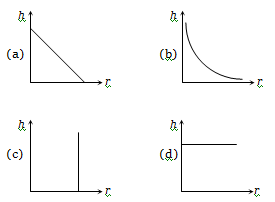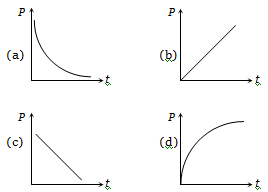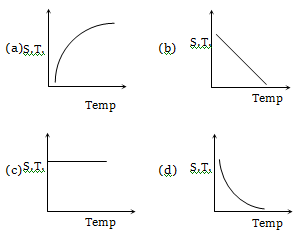Kerosene oil rises up the wick in a lantern

(a) Due to surface tension of the oil

(b) The wick attracts the kerosene oil

(c) Of the diffusion of the oil through the wick

(d) None of the above

Concept Questions :-

Properties of fluids

Difficulty Level:

Water rises against gravity in a capillary tube when its one end is dipped into water because

(a) Pressure below the meniscus is less than atmospheric pressure

(b) Pressure below the meniscus is more than atmospheric pressure

(c) Capillary attracts water

(d) Of viscosity

Concept Questions :-

Properties of fluids

Difficulty Level:

A capillary tube of radius R is immersed in water and water rises in it to a height H. Mass of water in the capillary tube is M. If the radius of the tube is doubled, mass of water that will rise in the capillary tube will now be

(a) M                                    (b) 2M
(c) M/2                                 (d) 4M

Concept Questions :-

Properties of fluids
High Yielding Test Series + Question Bank - NEET 2020

Difficulty Level:

There is a horizontal film of soap solution. On it, a thread is placed in the form of a loop. The film is pierced inside the loop and the thread becomes a circular loop of radius R. If the surface tension of the loop be T, then what will be the tension in the thread ?

(a) ${\mathrm{\pi R}}^{2}/\mathrm{T}$                      (b) ${\mathrm{\pi R}}^{2}\mathrm{T}$

(c) $2\mathrm{\pi RT}$                        (d) $2\mathrm{RT}$

Concept Questions :-

Properties of fluids
High Yielding Test Series + Question Bank - NEET 2020

Difficulty Level:

A large number of water drops each of radius r combine to have a drop of radius R. If the surface tension is T and the mechanical equivalent of heat is J, then the rise in temperature will be

(a) $\frac{2\mathrm{T}}{\mathrm{rJ}}$                                    (b) $\frac{3\mathrm{T}}{\mathrm{RJ}}$

(c) $\frac{3\mathrm{T}}{\mathrm{J}}\left(\frac{1}{r}-\frac{1}{R}\right)$                       (d) $\frac{2\mathrm{T}}{\mathrm{J}}\left(\frac{1}{r}-\frac{1}{R}\right)$

Concept Questions :-

Properties of fluids
High Yielding Test Series + Question Bank - NEET 2020

Difficulty Level:

In a surface tension experiment with a capillary tube, water rises upto 0.1 m. If the same experiment is repeated on an artificial satellite, which is revolving around the earth, water will rise in the capillary tube upto a height of-

(a) 0.1 m

(b) 0.2 m

(c) 0.98 m

(d) Full length of the capillary tube

Concept Questions :-

Properties of fluids
High Yielding Test Series + Question Bank - NEET 2020

Difficulty Level:

The correct curve between the height or depression h of liquid in a capillary tube and its radius isConcept Questions :-

Properties of fluids
High Yielding Test Series + Question Bank - NEET 2020

Difficulty Level:

A soap bubble is blown with the help of a mechanical pump at the mouth of a tube. The pump produces a certain increase per minute in the volume of the bubble, irrespective of its internal pressure. The graph between the pressure inside the soap bubble and time t will be-Concept Questions :-

Properties of fluids
High Yielding Test Series + Question Bank - NEET 2020

Difficulty Level:

Which graph represents the variation of surface tension with temperature over small temperature ranges for water?Concept Questions :-

Properties of fluids
High Yielding Test Series + Question Bank - NEET 2020

Difficulty Level:

In a capillary tube experiment, a vertical 30 cm long capillary tube is dipped in water. The water rises up to a height of 10cm due to capillary action. If this experiment is conducted in a freely falling elevator, the length of the water column becomes-
(a) 10 cm                             (b) 20 cm
(c) 30 cm                             (d) Zero

Concept Questions :-

Properties of fluids